# KSEEB SSLC Class 10 Maths Solutions Chapter 7 Coordinate Geometry Ex 7.3

In this chapter, we provide KSEEB SSLC Class 10 Maths Solutions Chapter 6 Constructions Ex 7.3 for English medium students, Which will very helpful for every student in their exams. Students can download the latest KSEEB SSLC Class 10 Maths Solutions Chapter 6 Constructions Ex 7.3 pdf, free KSEEB SSLC Class 10 Maths Solutions Chapter 6 Constructions Ex 7.3 book pdf download. Now you will get step by step solution to each question.

### Karnataka State Syllabus Class 10 Maths SolutionsChapter 6 Constructions Ex 7.3

Question 1.
Find the area of the triangle whose vertices are:
i) (2, 3), (-1,0), (2,-4)
ii) (-5, -1), (3, -5), (5, 2)
Solution:
i) Let A (2, 3) = (x1, y1)
B (-1, 0) = (x2, y2)
C (2, -4) = (x3, y3).
Area of the triangle from the given data: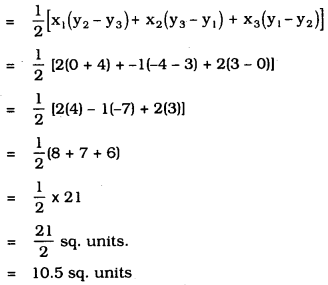∴ Area of ∆ ABC = 10.5 sq. units

ii) Let A (-5, -1) = (x1, y1)
B (3, -5) = (x2, y2)
C (5, 2) = (x3, y3).
Area of the triangle from the given data :∴ Area of ∆ ABC =32 sq. units

Question 2.
In each of the following find the value of ‘k’, for which the points are collinear.
i) (7, -2), (5, 1), (3, k)
ii) (8, 1), (k, -4), (2, -5)
Solution:
i) Let A (7,-2)= (x1, y1)
B (5, 1) = (x2, y2)
C (3, k) = (x3, y3).
Points are collinear, therefore the area of the triangle formed by these is zero (0).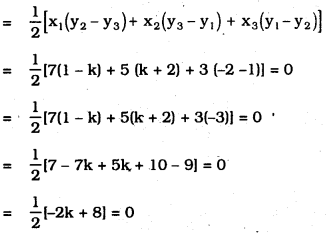-k + 4 = 0
-k = -4
∴ k = 4

ii) Let A (8, 1) = (x1, y1)
B (k, -4) = (x2, y2)
C (2, -5) = (x3, y3).
Area of Triangle ABC = 0
∴ ABC is a straight line.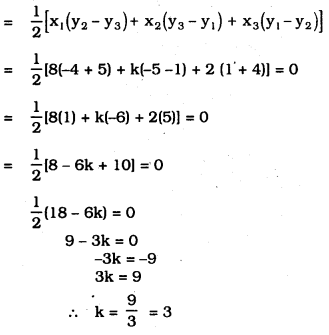Question 3.
Find the area of the triangle formed by joining the mid-points of the sides of the triangle whose vertices are (0, -1), (2, 1) and (0, 3). Find the ratio of this area to the area of the given triangle.
Solution:
Let the mid-points of ∆ABC are P, Q, R and also the mid-points of AB, BC and AC.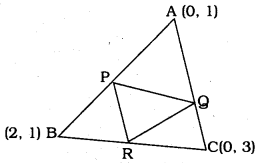As per Mid-point formula,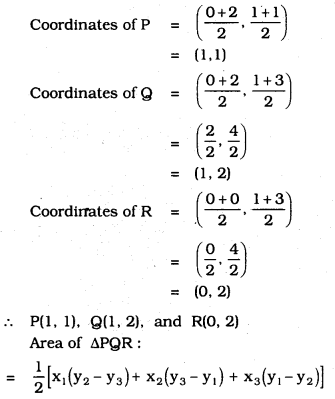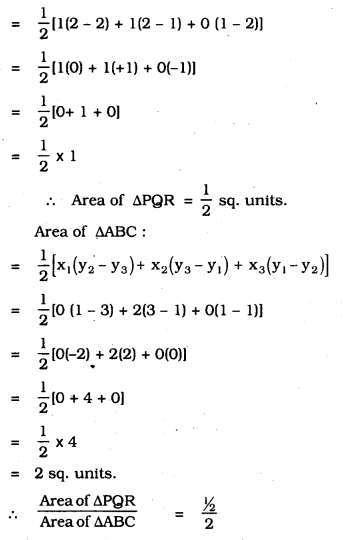=12×12=14
Area of ∆PQR : Area of ∆ABC
∴ 1 : 4

Question 4.
Find the area of the quadrilateral whose vertices, taken in order, are (-4, -2), (-3, -5), (3, -2) and (2, 3)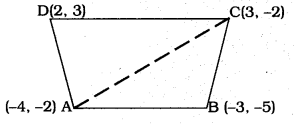Solution:
In the quadrilateral ABCD, diagonal AC is drawn which divides ∆ABC, ∆ACD.
Sum of these triangles is equal to the Area of the quadrilateral.
i) Now, Area of ∆ABC :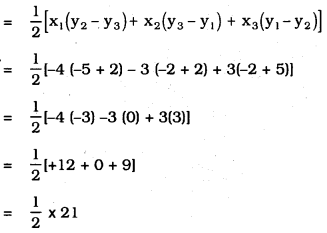= 10.5 sq. units

ii) Area of ∆ACD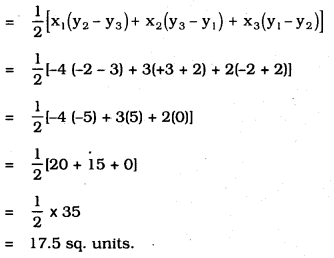= Area of ∆ABC + Area of ∆ACD = 10.5 + 17.5 = 28 sq. units.
∴ Area of quadrilateral ABCD = 28 sq.units.

Question 5.
You have studied in Class IX, (Chapter 9, Example 3), that a median of a triangle divides it into two triangles of equal areas. Verify this result for ∆ABC whose vertices are A(4, -6), B(3, -2) and C(5, 2).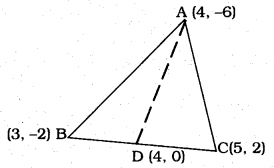Solution:
AD Median is drawn to side BC which is the vertex of ∆ABC.
Median AD divides AABC into two triangles ∆ABD and ∆ADC which are equal in area.
∴ BD = DC.
i) As per the Mid-Points formula,
Coordinates of D are
= (4)2,(0)2−−−−−−−√
= 16,0−−−−√
= (4,0)
∴ Coordinates of D are (4, 0)

ii) Now, Area of ∆ABD: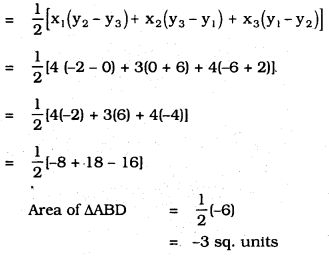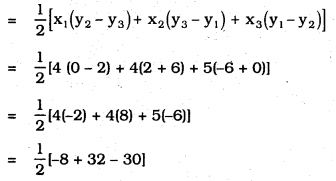= 12 × -6
= – 3 sq. units.
∴ Area of ∆ADC = -3 sq. units.
∴ Area of ∆ABC :
= Area of ∆ABD + Area of ∆ADC
= (-3) +(-3)
= -6 sq. units.

iv) Now, Area of ∆ABC : (Direct Method)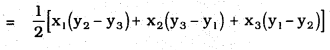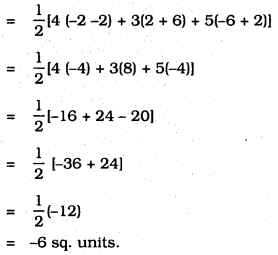All Chapter KSEEB Solutions For Class 10 Maths

—————————————————————————–

All Subject KSEEB Solutions For Class 10

*************************************************

I think you got complete solutions for this chapter. If You have any queries regarding this chapter, please comment on the below section our subject teacher will answer you. We tried our best to give complete solutions so you got good marks in your exam.

If these solutions have helped you, you can also share kseebsolutionsfor.com to your friends.

Best of Luck!!